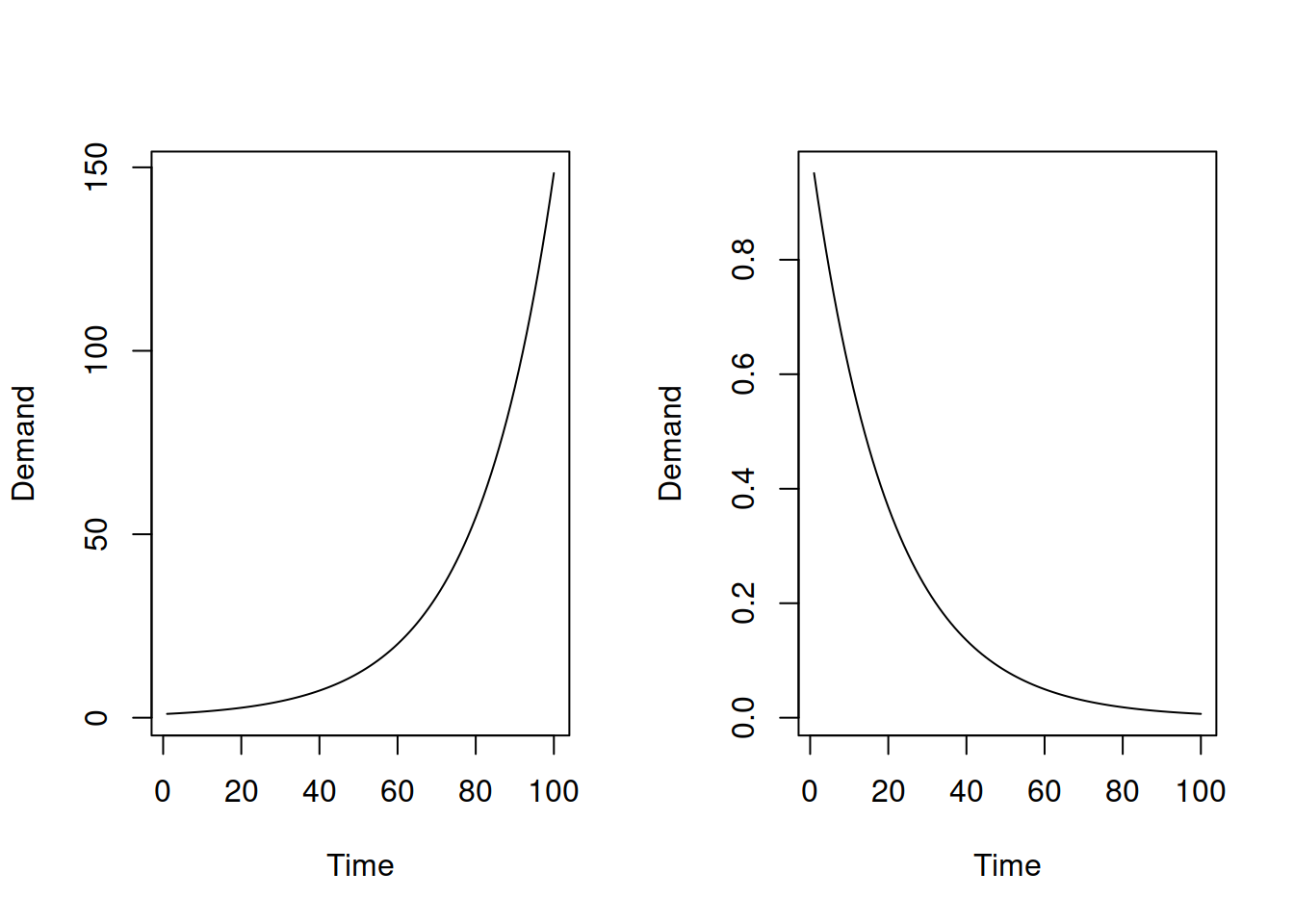This book is in Open Review. I want your feedback to make the book better for you and other readers. To add your annotation, select some text and then click the on the pop-up menu. To see the annotations of others, click the button in the upper right hand corner of the page

## 7.4 Mixed models with multiplicative trend

This is the most difficult class of models (MMA; MMdA; AMN; AMdN; AMA; AMdA; AMM; AMdM). These do not have analytical formulae for conditional moments, they are very sensitive to the values of smoothing parameters and may lead to explosive forecasting trajectories. So, in order to obtain conditional expectation, variance and prediction interval from the models of this classes, simulations should be used.

One of the issues that can be encountered, when using these models is the explosive trajectories because of the multiplicative trend. As a result, when these models are estimated, it makes sense to set the initial value of trend to 1 or a lower value, so that the optimiser does not encounter difficulties in the calculations.

Furthermore, the combination of components for the models in this class makes even less sense than the combination for the Class C (discussed in Section (ADAMETSMixedModelsGroup3)). For example, the multiplicative trend assumes either the explosive growth or decay as shown on the plots in Figure 7.1.Figure 7.1: Plots of exponential increase and exponential decline.

However, assuming that either seasonal component, or the error term, or both will have exactly the same impact on the final value irrespective of the point of time is unrealistic for these situation. The more reasonable would be for the amplitude of seasonality to decrease together with the exponential decay of the trend and for the variance of the error term to do the same. But this means that we are talking about the pure multiplicative models (Chapter 6.1), not the mixed ones. There is only one situation, where such mixed models could make sense: when the speed of change of the exponential trajectory is close to zero, meaning that the level of the series does not change rapidly and when the volume of the data is high. In this case the mixed models might perform well and even produce more accurate forecasts than the models from the other classes.

When it comes to the parameters bounds, this is a mystery for the mixed models of this class. This is because the recursive relations are complicated and calculating the discount matrix or anything like that becomes a challenging task. Using the usual bounds should still be okay, but keep in mind that these mixed models are typically not very stable, so from my experience the smoothing parameters should be as low as possible, assuming that the initial values guarantee a working model (not breaking at some of observations).